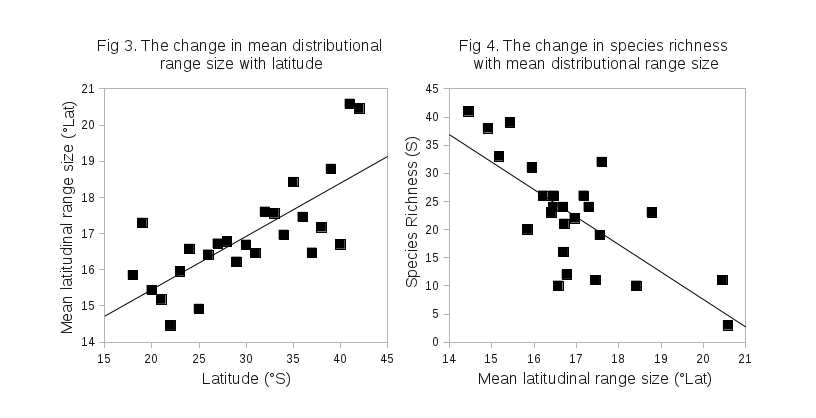# Distribution Range Size

The mean distribution range size of nematodes living in exposed sandy beaches increased with increasing latitude between 18 and 42 °S (Fig. 3). The mean distributional range size was calculated for each degree of latitude using the Stevens method. Pearson’s correlation (0.737, p < 0.001) and ‘ordinarly least squares’ regression analysis (R2 = 0.544, p < 0.001) indicated that the observed pattern was statistically significant. Futhermore, the species richness decreased with increasing mean distribution range size (Fig. 4). Pearson’s correlation (-0.732, p < 0.001) and ‘ordinarly least squares’ regression analysis (R2 = 0.536, p < 0.001) indicated that this pattern was also statistically significant.